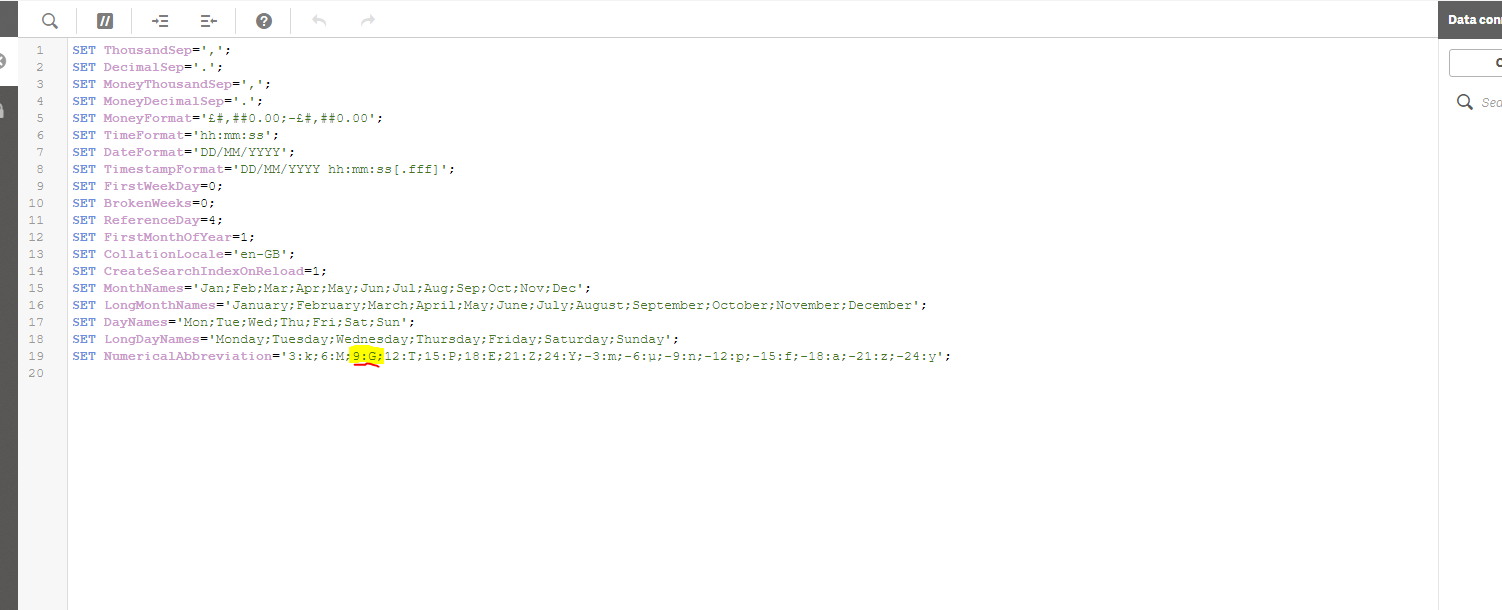New to Qlik Sense

Discussion board where members can get started with Qlik Sense.

AnnouncementsPartner

Formatting Numbers in a KPI - Qliksense

Hi There

I am using the same structured calculation as below and it works 100% in a flat table, as soon as you use it in a bar chart or KPI etc the numbers show but the symbol gets dropped for some reason.

if(Sum(Sales) > 1000000,  num(Sum(Sales)/1000000, '#.##0,##M', ',', '.'),

if(Sum(Sales) > 1000, num(Sum(Sales)/1000,  '#.##0,##K', ',', '.'), num(Sum(Sales), '#.##0,##', ',', '.')))

Using Auto or custom Formatting is not an option as I need to dictate to the calculation at what points I want what symbols.

Any ideas how to do this?

7 RepliesPartner

Re: Formatting Numbers in a KPI - Qliksense

Try

if(Sum(Sales) > 1000000,  num(Sum(Sales)/1000000, '#.##0M'),

if(Sum(Sales) > 1000, num(Sum(Sales)/1000,  '#.##0K'), num(Sum(Sales), '#.##0')))Partner

Re: Formatting Numbers in a KPI - Qliksense

The expression still gives me the same result but there is still no symbol in my KPI.

The issue is not the result its that the chart just decides on its own to exclude the symbols

Honored Contributor II

Re: Formatting Numbers in a KPI - Qliksense

=num(if(Sum(Sales) > 1000000,   Sum(Sales)/1000000 , '\$#,##0.00 M'),

num(if(Sum(Sales) > 1000,  Sum(Sales)/1000,   '\$#,##0.00 K'), num(Sum(Sales), '\$#,##0.00')))Partner

Re: Formatting Numbers in a KPI - Qliksense

Hi Aehman,

The calculation is perfect and works great for the Value but not for the custom symbol in a simple KPI chart or Bar chart.

I get the correct value eg if the answer is 535 000 000, with the calculation I get 535Mn in the flat table otherwise in the KPI chart I get 535 (Without the symbol).

The loss of the symbol in the chart is my issue.

Does that make sense?

Darryn

Honored Contributor II

Re: Formatting Numbers in a KPI - Qliksense

Maybe cus the expression was wrong?

I used

=if(Sum(Sales) > 1000000,  num(Sum(Sales)/1000000 , '\$#,##0.00 M'),

if(Sum(Sales) > 1000,  num(Sum(Sales)/1000,  '\$#,##0.00 K'), num(Sum(Sales), '\$#,##0.00')))

and it gives me K or M.

However you need to adjust number of 0 when you dividing the sum(Sale) to make sense of those symbols.HighlightedEmployee

Re: Formatting Numbers in a KPI - Qliksense

here is a simpler solution, just modify the symbol in the app set statement to anything you wantContributor II

Re: Formatting Numbers in a KPI - Qliksense

Hi @dvarner22 ,

Choice Measure expression :And insert the expression:

Dual(Num(Sum(Sales)/Pow(10,3*(Div(Log10(Sum(Sales)),3))),'#.##0,0')&pick(div(Log10(Sum(Sales)),3),'k','M','B','T'),Sum(Sales))

I hope helps.